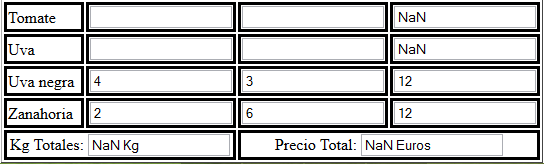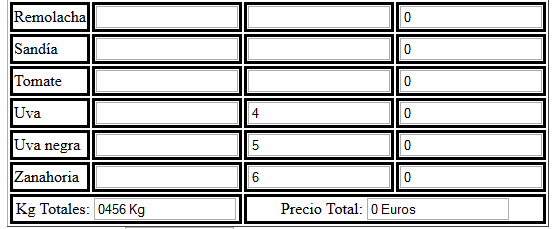# sevillano665

Members

7

## Posts posted by sevillano665

1. ### "Require_once" produces "undefined variable"

Hi guys! I have a little problem and it's that I get an error in a determined function where I call my connection and I don't know why. The errors are:

1) Notice: Undefined variable: conexionidiomas in C:\wamp64\www\idiomas\preguntas-frecuentes.php on line 10
2) Warning: mysqli_real_escape_string() expects parameter 1 to be mysqli, null given in C:\wamp64\www\idiomas\preguntas-frecuentes.php on line 11
This is what I have from "/Connections/conexionidiomas.php":
```# FileName="Connection_php_mysql.htm"
# Type="MYSQL"
# HTTP="true"
\$hostname_conexionidiomas = "p:localhost:3307";
\$database_conexionidiomas = "idiomasbd";
```

and this in "preguntas-frecuentes.php":

```<?php require_once('Connections/conexionidiomas.php'); ?>
<?php
if (!function_exists("GetSQLValueString")) {
function GetSQLValueString(\$theValue, \$theType, \$theDefinedValue = "", \$theNotDefinedValue = "")
{
if (PHP_VERSION < 6) {
\$theValue = get_magic_quotes_gpc() ? stripslashes(\$theValue) : \$theValue;
}

\$theValue = function_exists("mysqli_real_escape_string") ? mysqli_real_escape_string(\$conexionidiomas,
\$theValue) : mysqli_escape_string(\$conexionidiomas,\$theValue);

switch (\$theType) {
case "text":
\$theValue = (\$theValue != "") ? "'" . \$theValue . "'" : "NULL";
break;
case "long":
case "int":
\$theValue = (\$theValue != "") ? intval(\$theValue) : "NULL";
break;
case "double":
\$theValue = (\$theValue != "") ? doubleval(\$theValue) : "NULL";
break;
case "date":
\$theValue = (\$theValue != "") ? "'" . \$theValue . "'" : "NULL";
break;
case "defined":
\$theValue = (\$theValue != "") ? \$theDefinedValue : \$theNotDefinedValue;
break;
}
return \$theValue;
}
}

\$editFormAction = \$_SERVER['PHP_SELF'];
if (isset(\$_SERVER['QUERY_STRING'])) {
\$editFormAction .= "?" . htmlentities(\$_SERVER['QUERY_STRING']);
}

if ((isset(\$_POST["MM_insert"])) && (\$_POST["MM_insert"] == "form1")) {
\$insertSQL = sprintf("INSERT INTO tblfrecuentes (strPregunta,fchFecha) VALUES (%s,NOW())",GetSQLValueString(\$_POST['strPregunta'], "text"));

\$Result1 = mysqli_query(\$conexionidiomas,\$insertSQL) or die(mysqli_error(\$conexionidiomas));
}
?>
```
So, if someone could help me I'd appreciate it so much. Regards!
2. ### HashCode for calculate different objects

Hello, I'm trying to make a method that creates objects of a parameterized type randomly, but also to store the hashCode of different objects created and if at any time the percentage of different objects is less than 50% throw an exception.
This last part is where I've gotten stuck. I have created a population property where I store the different hashCodes and update it in the method adding the new hashCode from the new object. But I don't know how to do for to know if the percentage of different objects is less than 50%. See if someone can help me out.
Regards!
3. ### "var" doesn't work as number

There's parseFloat instead. Both parseInt and parseFloat will return NaN (not a number) if the value could not be converted to a number. So, it sounds like the values in those fields are not numeric values. You can use the isNaN function to check if a value is NaN. If it is NaN, then show an error message that they have to enter a numeric value.

But I really want that the result text boxes of every multiplication contain "0" if I don't introduce any number, in this way the sum will be made. I have tried to put by default a "0" in the result text boxes but when I introduce some number in some text box all result text boxes shows "NaN" and the sum is not made.

4. ### "var" doesn't work as number

Then show your updated code. If it isn't working then you didn't do it right. There are examples of using things like parseInt online if you want to research that.

Here is:

`<script type="text/javascript">           function multiplicar() {          	var contador1;          	var contador2 = 2;          	var contador3 = 1;          	var n1;          	var n2;           	var id1;          	var id2;          	var id3;          	var kgTotales = 0;          	var precioTotal = 0;          	          	for(contador1=1; contador1 < 60; (contador1)+2){          		id1="n" +(contador1);          		id2="n" +(contador2);          		id3="result" +(contador3);          		          		contador1=(contador1)+2;          		contador2=(contador2)+2;          		contador3++;          		          		          		          		n1=parseFloat(document.getElementById(id1).value);          		          		n2=parseFloat(document.getElementById(id2).value);                        document.getElementById(id3).value = (n1*n2);          	          	          	precioTotal = (precioTotal) + (n1*n2);              	          	kgTotales = (kgTotales)+n2;          	   	          	          	document.getElementById("precioTotal").value = precioTotal + " Euros";          	document.getElementById("kgTotales").value = kgTotales + " Kg";}}</script>`

And this is the view, the column where are 3 and 6 are "kg" and its sum have to be shown in kgTotales box, and the last one is for the price.Regards!

5. ### "var" doesn't work as number

if you expect them to be whole numbers, I would use parseInt, with a base 10 radix

`parseInt("2", 10);`

It doesn't work, the same result

kgTotales is already a number. The strings you need to convert to numbers are n1 and n2.

I realized it and I changed, but the result is the same too :S

6. ### "var" doesn't work as number

Are you checking for errors in your console? What debugging are you doing? You should trace the value of precioTotal in your script and make sure you are getting the right values you expect.

`console.log('precioTotal before => ' + precioTotal);console.log('n1 is => ' + n1 + ', n2 is => ' + n2);precioTotal = precioTotal + (n1*n2);console.log('precioTotal after => ' +  precioTotal);`

The table multiply the 2nd column and the third one and the result is shown in the fourth, when I execute and write the values, the result is correct, so it's not possible there's some error en n1 or n2 I think, and precioTotal shows the correct result too. I think I'm doing something wrong and it doesn't recognize the var as a string.

Any time you get a value from a form element or anything else on the page, the value is always a string. You can use functions like parseInt, parseFloat, or Number to convert to a number.

Like this?

`parseFloat(kgTotales);kgTotales = (kgTotales+n2);`
7. ### "var" doesn't work as number

Hi! My problem is that I've made a function with "var kgTotales = 0". But when I sum "kgTotales = kgTotales + 2" the result is "02", it returns a string and not a number. I use this var in a bucle so the result is "kgTotales = 02...." and next to it the next numbers I want to sum.

This is the code:

`<script type="text/javascript">           function multiplicar() {          	var contador1;          	var contador2 = 2;          	var contador3 = 1;          	var n1;          	var n2;           	var id1;          	var id2;          	var id3;          	var kgTotales = 0;          	var precioTotal = 0;          	          	for(contador1=1; contador1 < 60; (contador1)+2){          		id1="n" +(contador1);          		id2="n" +(contador2);          		id3="result" +(contador3);          		          		contador1=(contador1)+2;          		contador2=(contador2)+2;          		contador3++;          		          		          		          		n1=document.getElementById(id1).value;          		          		n2=document.getElementById(id2).value;          		          			          	     	     	          	          	        	          	document.getElementById(id3).value = (n1*n2);          	          	          	precioTotal = precioTotal + (n1*n2);              	          	kgTotales = kgTotales+n2;      	          	          	document.getElementById("precioTotal").value = precioTotal + " Euros";          	document.getElementById("kgTotales").value = kgTotales + " Kg";          	          	          	          	          	          	}          	          	          	                  	          	} </script> `

And this is the result:I don't understand why it happens because precioTotal is a similar var and also shows a sum and works well. I hope someone could helps me.

Regards!

×